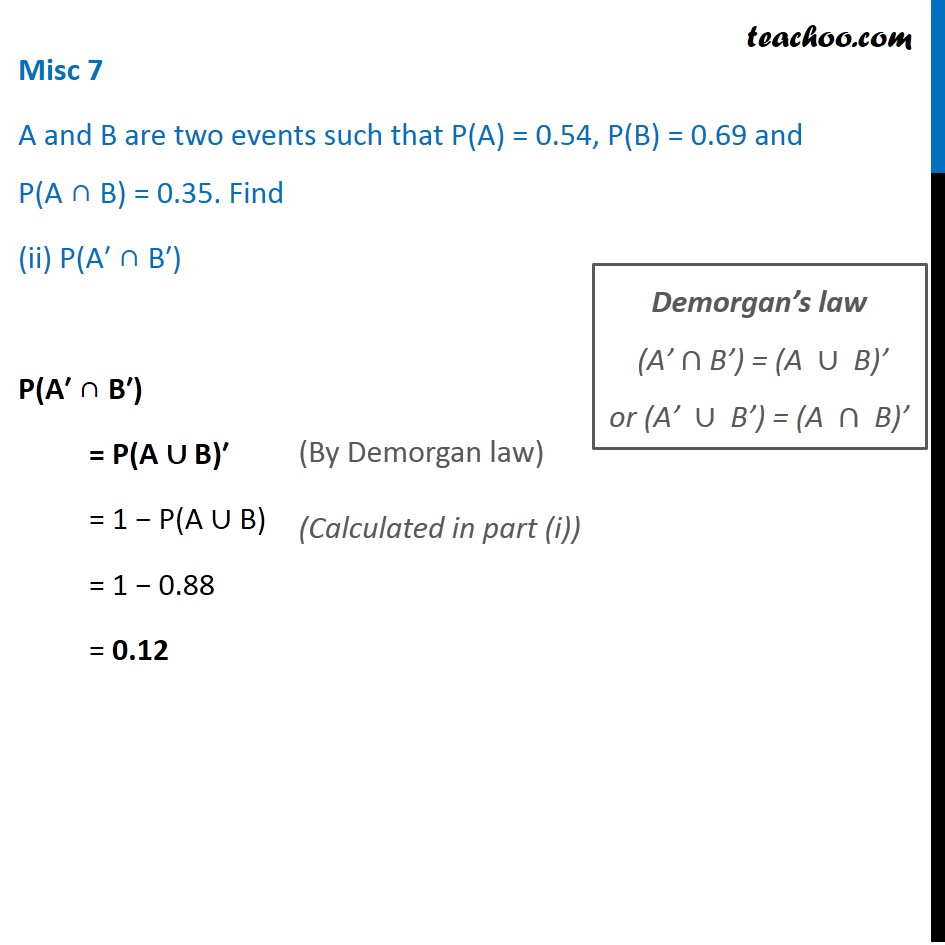Miscellaneous

Chapter 14 Class 11 Probability
Serial order wiseLearn in your speed, with individual attention - Teachoo Maths 1-on-1 Class

### Transcript

Misc 7 A and B are two events such that P(A) = 0.54, P(B) = 0.69 and P(A ∩ B) = 0.35. Find (ii) P(A′ ∩ B′) P(A′ ∩ B′) = P(A ∪ B)′ = 1 − P(A ∪ B) = 1 − 0.88 = 0.12 (By Demorgan law) Demorgan’s law "(A’"∩"B’) = (A "∪" B)’" "or (A’ "∪" B’) = (A "∩" B)’"